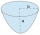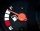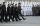# Functions - math word problems

#### Number of problems found: 66

• Degrees Fahrenheit? The equation above shows how temperature F, measured in degrees Fahrenheit, relates to a temperature C, measured in degrees Celsius. Based on the equation, which of the following must be true? I. A temperature increase of 1 degree Fahrenheit is equivale
• Eq2 2Solve following equation with quadratic members and rational function: (x2+1)/(x-4) + (x2-1)/(x+3) = 23
• Find the 15Find the tangent line of the ellipse 9 x2 + 16 y2 = 144 that has the slope k = -1
• The parabolic segmentThe parabolic segment has a base a = 4 cm and a height v = 6 cm. Calculate the volume of the body that results from the rotation of this segment a) around its base b) around its axis.
• College 2College student is moving into a dormitory. The student rent a truck for \$19.95 plus \$0.99 per mile. Before returning the truck the student fills the tank with gasoline, which cost \$65.32. Total cost \$144.67. Using a linear equation, explain the process tUsing one of the following forms x+p=q or px=q write an to represent these problems using x as the unknown variable Emily can jump twice as far as Evan on the broad standing board if Emily can jump 6.5 feet. How many feet can Evan jump?